## 19.2. Constructing tables

In this section we will introduce the basic concepts of tables and explain the basic formatting possibilities that are available.

### 19.2.1. Basic tables

A table is created as an instance of the `class GTextTable` and can be managed in much the same way as for example Icons, Texts or other plot objects. It can be added to the graph (at specified X and Y coordinates) using the standard `Graph::Add()` method.

The creation of a table starts with including the necessary file "`jpgraph_table.php`" which contains the class definition for the `GTextTable` class. In order to create a table we also need a graph context to which the table can be added. This can be either one of the ordinary graphs which used an instance of `class Graph` or to create a stand alone table by using an instance of `class CanvasGraph`

Creating a new table is then just matter of creating a new instance of the `class GTextTable` and calling the initialization method with the desired size (in rows and columns) of the table. To display the table stand alone we also need to create a canvas graph and then add the table to the graph.

 ```1 2 3 4 5 6 7 8 9 10 11 12 13 14 15 16 17 18 19 20 ``` ```Init(5,7); // Create a 5 rows x 7 columns table ... // Add the table to the graph \$graph->Add(\$table); // and send back to the client \$graph->Stroke(); ?>```

As can be seen above it is not necessary (but still possible) to specify an explicit physical size for the table. The physical size of the table will be automatically determined depending on the content of the table.

The cells in the table are numbered sequentially where (0,0) is the top left and (n-1, m-1) is the bottom right cell in a table with n rows and m columns.

The next step is to populate the table with some data. The content in one cell is specified with the using one of the following methods

• `GTextTable::Set(\$aRow, \$aCol, \$aText)`

Set the specified text in cell (`\$aRow`, `\$aCol`)

`GTextTable::Set(\$aData)`

Copy the data in the two dimensional array `\$aData` to the table

• ```GTextTable::SetImage(\$aRow, \$aCol, \$aText, \$aImage)```

Set the specified image in cell (`\$aRow`, `\$aCol`)

• `GTextTable::SetCellCountryFlag(\$aRow,\$aCol,\$aFlag,\$aScale=1.0,\$aMix=100,\$aStdSize=3)`

Set the specified country flag in cell (`\$aRow`, `\$aCol`)

The `Set()` method is polymorphic and can be called in two different ways.

It can either be used to specify the value of each individual cell by identifying the cell by its row and column index (starting at 0) ways. For example to continue the example above we set the first two cells on the first row to 1 and 2 vi the two calls

 ```1 2 3 4 ``` ```... \$table->Set(0,0,1); \$table->Set(0,1,1); ...```

The second, and probably less tedious way is to setup a 2-dimensional array (matrix) with suitable values and then pass that 2-dimensional array as the first and only argument to the `Set()` method as the following example shows

 ```1 2 3 ``` ```\$data = array( array( 12, 7), array( 10, 5)); \$table->Set(\$data)```

If the table is specified by a matrix directly it is not necessary to make the initial `Init()` call since it will be automatically determined by the size of the matrix.

The following basic full example creates a 2 rows by 4 columns table with consecutive values. Note here that we make use of `CanvasGraph` to provide the graph on which to add the table. The result of this script is shown in Figure 19.3

Example 19.1. The most basic 2x4 table (`table_howto1.php`)

 ```1 2 3 4 5 6 7 8 9 10 11 12 13 14 15 16 17 18 19 20 ``` ```Set(\$data); // Add the table to the graph \$graph->Add(\$table); // ... and send back the table to the client \$graph->Stroke(); ?>```

Figure 19.3. The most basic 2x4 table `(table_howto1.php)`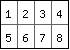In the example above we also made use of a minor shortcut. If the data to the table is specified with a 2-dimensional array there is no need to call the `Init()` method since the size will be determined by the supplied array.

We will not yet begin discussion how to change fonts, colors and grids. Instead we will focus on the structure of the table and introduce the merge operation.

### 19.2.2. Merging cells

The only way to change the structure (as opposed to the look & feel) of the table is by merging two or more cells. The merge operation is done via one of three method calls:

• `MergeRow()`

Merge all cells in a specified row

• `MergeCol()`

Merge all cells in a specified column

• `MergeCells()`

Merge a rectangular range of cells

The first two methods are really just simplifications for the third most generic method. The first two methods merge all the cells in a specified row or column and the third method merges any arbitrary rectangular range of cells specified by the upper left nd lower right corner.

In addition, by default, these calls will also center align the text in the merged cells (both horizontally and vertically). This can however be adjusted by giving the wanted alignment as additional argument to the methods or by calling the specific APIs that controls the alignment of the data within each cell.

The third (and most generic) method merge a range of cells specified by the top left and bottom right corner. So, for example, the following line will merge the rightmost four cells in the previous example

 ```1 ``` `\$table->MergeCells(0,2,1,3);`

Figure 19.4. Merging the rightmost 4 cells in the table `(table_howto2.php)`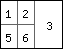The merged cell can later on be referenced by the top left of the original merged cell range. This means that the merged cell in the above example is referred to as the (0,2) cell.

If we instead wanted to merge the top row of the table we could have simplified the call by using one of the alternative merge methods. In this case the``` MergeRow()``` method would be applicable as in

 ```1 ``` `\$table->MergeRow(0);`

which would then give the table as shown in Figure 19.5

Figure 19.5. Merging the top row `(table_howto3.php)`In summary, the following three rules apply to merging and/or merged cells

• It is an error to try o merge already merged cells.

• A merged cell is referenced by the top left cell in the merged range.

• All formatting that can be applied to a single cell can also be applied to a merged cell.

The most basic way to change the look and feel of the table is to adjust the font that is used in the different cells in the table. To adjust the font within the table the following methods are available

• `SetFont()`, Specify the default font for the entire table or for a specified range of cells

• `SetRowFont()`, Specify the font for an entire row of cells

• `SetColFont()`, Specify the font for an entire column of cells

• `SetCellFont()`, Specify the font for a single cell

By default the font in the table is set to `FF_FONT1`, i.e. the built-in bit mapped font of medium size. The default color of the font is 'black'.

### Note

If several font settings are applied to the same cell it will be the last method call before the table `Stroke()` method that will take precedence.

As a simple example the following script shows how to adjust the font used in the first merged row in the previous example.

Figure 19.6. Adjusting the font in the top row `(table_howto5.php)`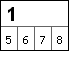The font in Figure 19.6 was adjusted with a call to

 ```1 ``` `\$table->SetCellFont(0,0,FF_ARIAL,FS_BOLD,14);`

Polymorphic `SetFont()`

Before finishing this section it is worth noting that the `SetFont()` method is polymorphic and can be called in two different ways.

1. In the first way it can be called with a single font (triple) argument. That font specification will then become the default for all cells in the table, for example

 ```1 ``` `\$table->SetFont(FF_TIMES,FS_NORMAL,12);`

will set the entire table to use Times TTF font.

2. In the second way the first four arguments specifies a cell range (top left, bottom right) to apply the font setting to, for example

 ```1 ``` `\$table->SetFont(0,1,1,2,FF_TIMES,FS_NORMAL,12);`

In order to adjust the look and feel of the table it is possible to set the following colors of the table:

• The color of the text/number in each cell

• The background color of each cell

• The color of the grid (inside the table) and border (around the table)

The font color for a cell (or range of cells) is specified with one of

• `SetColor()`, Set the color for a range of cells or the entire table

• `SetRowColor()`, Set the color for a specified row of cells

• `SetColColor()`, Set the color for a specified column of cells

• `SetCellColor()`, Set the color for a specified cells

In a similar way the background color (or the fill color) of a cell can be specified with

• `SetFillColor()`, Set the fill color for a range of cells or the entire table

• `SetRowFillColor()`, Set the fill color for a specified row of cells

• `SetColFillColor()`, Set the fill color for a specified column of cells

• `SetCellFillColor()`, Set the fill color for a specified cells

The way to adjust the color of the grid and border will be discussed in the section regarding borders and grids below.

Polymorphic `SetColor()` and `SetFillColor()`

In the same manner as `SetFont()` can act on both all cells and a specified range of cells the two color setting methods work in the same way. Both `SetColor()` and `SetFillColor()` can both be called either with a single argument (in which all cells will be addressed) or by giving a range (top left and bottom right cell) to be acted upon.

The following method calls will set the background color of the first three cells in row 2 and 3 and the entire table

 ```1 2 3 4 5 6 7 ``` ```... // First set the default fill color to lightgray \$table->SetFillColor('lightgray'); // The Set a range to yellow \$table->SetFillColor(2,0,3,2,'yellow'); ...```

Finally we give some concluding examples to make the usage of these methods more clear.

 ```1 2 3 4 5 6 7 8 9 10 11 ``` ```... // Set the default font color for all table cells \$table->SetColor('darkgray'); // Set the font color for all cells in row one \$table->SetRowColor(1,'darkgray'); // Set the font color for the first two cells in the second row // (row with index=1) \$table->SetColor(1,0,1,1,'darkgray'); ...```

### Note

If there are multiple color settings for the same cell then the last method called before the final Stroke() call will be the color that shows.

For example the following script sets the color of cell (0,0) to both 'black' and 'yellow' but since the yellow is the last method to be called this is the color that will be used for cell (0,0)

 ```1 2 3 4 5 ``` ```... \$table->SetRowColor(0,'black'); \$table->SetColColor(0,'yellow'); ... \$graph->Stroke();```

Finally, we illustrate the usage of these method by continuing with the previous example and coloring row 0 as shown in Figure 19.7

Figure 19.7. Merging and setting the colors the top row `(table_howto4.php)`The coloring in Figure 19.7 was accomplished by adding the following two lines to the previous script.

 ```1 2 ``` ```\$table->SetCellFillColor(0,0,'orange@0.7'); \$table->SetCellColor(0,0,'darkred');```

The same effect could also have been accomplished by using the row version of the color methods as in

 ```1 2 ``` ```\$table->SetRowFillColor(0,'orange@0.7'); \$table->SetRowColor(0,'darkred');```

Which sets of methods to use in this case is a matter of personal preference since both achieve the same effect.

### 19.2.5. Adjusting table and cell sizes

The exact size of the table can only be indirectly controlled by specifying the width of the columns and the height of the rows. The width/height can either be controlled individually on a row by row (or column by column) basis or by giving all rows/columns the same width/height. This is accomplished by the methods

• `SetMinRowHeight()`, Sets the minimum height of rows

• `SetMinColWidth()`, Sets the minimum width of columns

As can be noted from the name of the methods the width and height specified is not necessarily the exact size of the row/column. It's just the minimum size. The actual size is depending on the text in the cells. The row height (and column width) will always grow in order to fit the text within the largest cell.

Polymorphic functions

The number of arguments to the two methods can in fact be either one or two. If only one argument is supplied then it is interpretated as the minimum width/height for all columns/rows in the table. If two arguments are used then the first argument will specify what row/columns the height/width is specified for.

Continuing with the table in Figure 19.7 . If we want to make sure that all the cells in row 1 is at least 35 pixels wide we could add to add the following in the previous script

 ```1 2 3 ``` ```... \$table->SetMinColWidth(35); ...```

Which would then give the table shown in Figure 19.8

Figure 19.8. Setting the minimum column width to 35 pixels. `(table_howto6.php)`### 19.2.6. Fine tuning cell alignment and cell padding

The final bit of important formatting is the ability to specify the alignment of the data in each of the cells within the table. It is possible to specify both the horizontal and vertical alignment. The alignment can be specified as one of

• Horizontal alignment: "left", "right", "center"

• Vertical alignment: "top", "bottom", "center"

As usual there are four variants of the alignment methods

• `SetAlign()`, Set the align for a range of cells or the entire table

• `SetRowAlign()`, Set the alignment for an entire row

• `SetColAlign()`, Set the alignment for an entire column

• `SetCellAlign()`, Set the alignment for a specific cell

Each of the methods accepts two alignment arguments, the horizontal and the vertical alignment. We don't give any examples here since the usage of these methods should be obvious.

### Note

It should be mentioned that in addition to using these methods the alignment can also be set when using the merge methods (as discussed above). This is purely as a convenience since it is such a common use case to adjust the alignment when merging cells.

### 19.2.7. Adjusting border and grid lines

The final formatting option available is the shape, size and color of the border and grid lines in the table. As of this writing the library supports the following styles of grid lines.

• `TGRID_SINGLE`, a basic solid line of specified width and color (default)

• `TGRID_DOUBLE`, two lines of equal width separated by the same width as the line width.

• `TGRID_DOUBLE2`, two lines where the left/top line is twice as thick as the bottom/right line.

 Figure 19.9. Double lines 1 `(table_howto7.1.php)`Figure 19.10. Double lines 2 `(table_howto7.2.php)`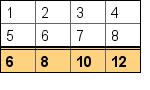The methods available to adjust the grid lines are

• `SetGrid()`, Set the grid line style for all grid lines

• `SetColGrid()`, Set the specified vertical grid line

• `SetRowGrid()`, Set the specified horizontal grid line

The borders in Figure 19.9 was modified by adding the following call to the basic table script.

 ```1 ``` `\$table->SetRowGrid(2,1,'black',TGRID_DOUBLE);`

and in Figure 19.10

 ```1 ``` `\$table->SetRowGrid(2,1,'black',TGRID_DOUBLE2);`

The order of the arguments are 1) Row, 2) Width (weight) and 3) Line style.

Finally, in order to adjust the outer border of the table there is one last method available

• `SetBorder()`, Set the width and color of the outer border

### Tip

In order to remove a specific grid line or the border the width is specified as 0

As a final example the following script snippet removes many of the grid lines and borders by adding the following lines to the previous basic table script.

 ```1 2 3 4 5 ``` ```... \$table->SetBorder(0); \$table->SetGrid(0); \$table->SetRowGrid(2,1,'black',TGRID_DOUBLE2); ...```

The result of this modification is shown in Figure 19.11 below.

Figure 19.11. Removing some grid lines and border. In addition we have right aligned all the cells as is common practice for numeric data. `(table_howto8.php)`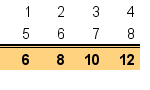### 19.2.8. Specific number formatting

The final method of formatting we will briefly touch upon is the number formatting. By setting a specific number formatting it is possible to have numeric values formatted in a uniform way regardless of the original format. The number format is the same format string that would be used with the `printf()` family.

The available methods for this formatting follows the usual structure

• `SetNumberFormat()`, Set the number format for a range or the whole table

• `SetRowNumberFormat()`, Set the number format for a specific row

• `SetColNumberFormat()`, Set the number format for a specific column

• `SetCellNumberFormat()`, Set the number format for a specific cell

To illustrate the use of this formatting we start with the table in Figure 19.11. and apply the format string `"%0.1f"` which will format the values as floating point values with one decimal. We do this by adding the method following method call

 ```1 ``` `\$table->SetNumberFormat('%0.1f');`

Figure 19.12. Applying a number format to the data in the cells `(table_howto9.php)`### Note

The number format will only set the number format it will not do any rounding of the actual values. This is the responsibility of the client.

### 19.2.9. Using images and country flags in the table

In addition to ordinary text it is also possible to have arbitrary images (read from a file) or use one of the built-in country flags available in the library as background images in the table cells.

### Note

Remember to include "`jpgraph_iconplot.php`" if images should be included and "`jpgraph_flags.php`" if country flags are included.

The methods available for this purpose are

• `SetImage()`, Set the same image for all cells or a range of cells

• `SetCellImage()`, Set the image for a specific cell

• `SetRowImage()`, Set the image for a row of cells

• `SetColImage()`, Set the image for a column of cells

• `SetCellImageConstrain()`, Set the height or width of an image in a specified cell

• `SetCellCountryFlag()`, Use a specified country flag as image in a specified cell

For example, to add the United Kingdoms flag in cell (2,1) in the table one would have to add the call

 ```1 ``` `\$table->SetCellCountryFlag(2,1,'united kingdom');`

as usual with the background images it is possible to scale it and also adjust the mix-in factor by using additional arguments to the above methods.

However there is on extra feature available with images and tables. If we wanted to make sure that the flag is of a specific height or width it is possible to specify this and have the library auto-scale the image to fit the specified parameter.

For example to restrict the height of the UK flag set in cell (2,1) to 20 pixels the call would be

 ```1 ``` `\$table->SetCellImageConstrain(2,1,TIMG_HEIGHT,20);`

We conclude this section with a small example on the use of country flags in a table in Figure 19.13

Figure 19.13. Using country flags in the table cells `(table_flagex1.php)`